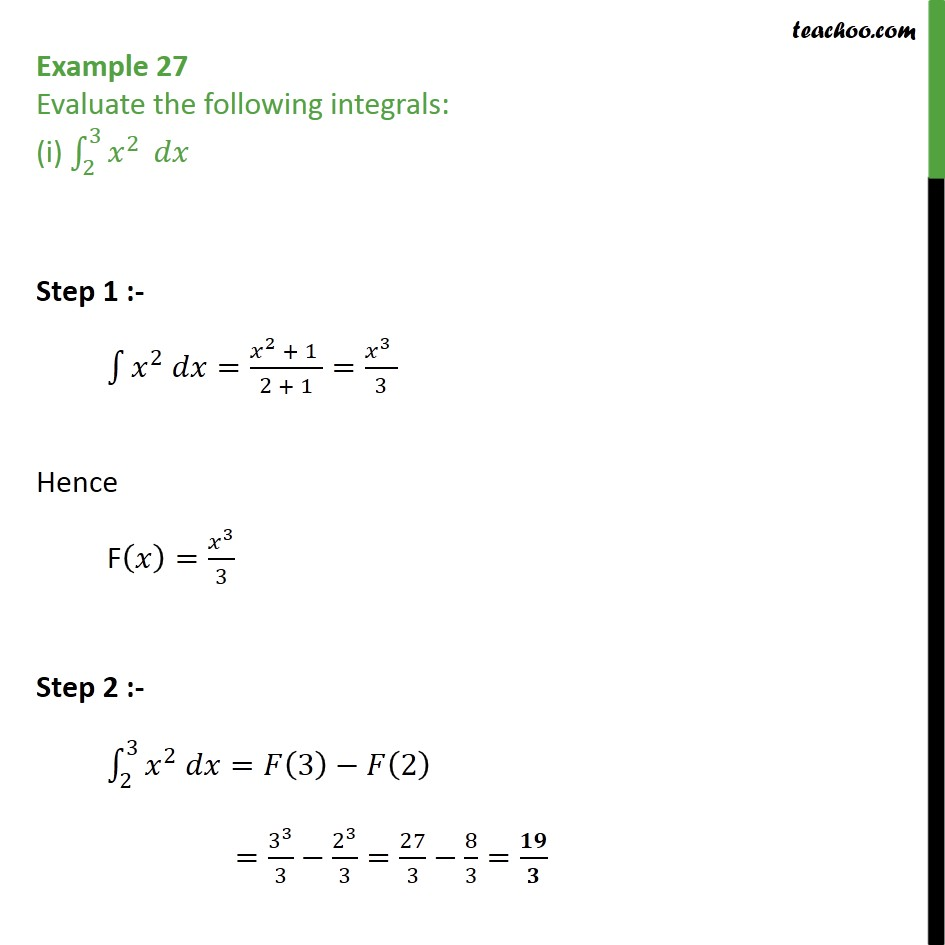Definite Integration - By Formulae

Chapter 7 Class 12 Integrals
Concept wiseLearn in your speed, with individual attention - Teachoo Maths 1-on-1 Class

### Transcript

Example 27 Evaluate the following integrals: (i) 2 3 2 Step 1 :- 2 = 2 + 1 2 + 1 = 3 3 Hence F = 3 3 Step 2 :- 2 3 2 = 3 2 = 3 3 3 2 3 3 = 27 3 8 3 = 19 3